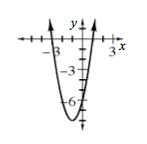### Home > A2C > Chapter 1 > Lesson 1.2.2 > Problem1-90

1-90.

Make a complete graph of the function $h(x) = 2x^2 + 4x − 6$ and describe its domain and range.

Enter different $x$-values into the equation and observe the behavior of the $y$-values of the function, as well as the points on the graph.

For the domain, what possible $x$-values can you enter into the equation?

$x$-values greater than $2$ or less than $−3$ will give $y$-values greater than $9$.

What is the smallest $y$-value? Is there a largest? What is the range?

What are the $x$- and $y$-intercepts?

Is the graph symmetric? What is the line of symmetry? Is there a highest point? A lowest?Use the eTool below to make a complete graph of the function.
Click the link at right for the full version of the eTool: A2C 1-90 HW eTool